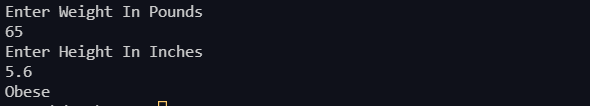## BMI Calculator

Write a Java application with the following prototypes that returns the user's body mass index (BMI)

public static double calcluateBMI(double weight, double height)

To calculate BMI based on weight in pounds (lb) and height in inches (in), use this formula:

and

public static String findStatus(double bmi)

Categorizes it as underweight, normal, overweight, or obese, based on the table from the United States Centers for Disease Control:

 BMI Weight Status Below 18.5 Underweight 18.5 – 24.9 Normal 25.0-29.9 Overweight 30.0 and above Obese

Prompt the user to enter weight in pounds and height in inches.

import java.util.Scanner;
public class CalculateBMI {
public static double calculateBMI(double weight, double height)
{
double bmi = (weight*703)/ height * height;
return bmi;
}
public static String findStatus(double bmi){
if (bmi < 18.5)
{
return "Underweight";
}
else if (bmi >= 18.5 && bmi < 24.9)
{
return "Normal";
}
else if (bmi >= 24.9 && bmi < 29.9)
{
return "Overweight";
}
else
{
return "Obese";
}
}
public static void main(String [] arg){
Scanner user_input = new Scanner(System.in);
System.out.println("Enter Weight In Pounds ");
double weight = user_input.nextDouble();
System.out.println("Enter Height In Inches ");
double height = user_input.nextDouble();
double bmi = calculateBMI(weight, height);
System.out.println(findStatus(bmi));

}
}java programming exercise with solution (methods)